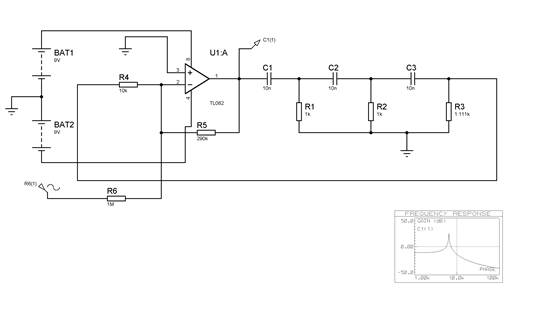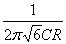# In Phase Shift RC Oscillator Assignment HelpAs before the simulation of the oscillator will not start on its own. This time we are applying an input, which is a sine generator via R6 (1M).

The analysis is performed with a frequency graph (see fig 19).Figure 19

To set up the analysis, draw out a frequency graph and then click onto the sine generator, drag and place onto the horizontal axis of the graph. The frequency of the generator is now controlled by the analysis. Now click onto the output voltage probe and drag it to the vertical axis of the graph.

## In Phase Shift RC Oscillator Assignment Help Through Online Tutoring and Guided Sessions from AssignmentHelp.Net

Set the properties of the graph to run from 1kHz to 100kHz.

Perform the simulation and examine the output.

The oscillator is highly resonant at a frequency ofWith the values set at R = 1k and C = 10n, check that resonance occurs at the correct frequency.

Re-run the simulation with R = 1k and C = 1n. Comment on your results. Why does the frequency plot roll off as the frequency goes above resonance?

Assignments In Phase Shift RC Oscillator | Physics | Study Physics | Physics Online Tutor | Physics Homework Help | Physics Help | Physics Solutions | Physics Problems | Physics Tutor | Physics Online Tutoring | Tutoring Services | Private Tutors | Writing Help | Term Paper Help | Sample Homework | Online Tutoring